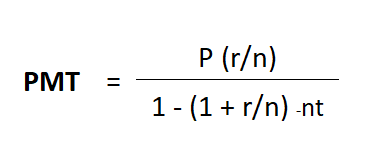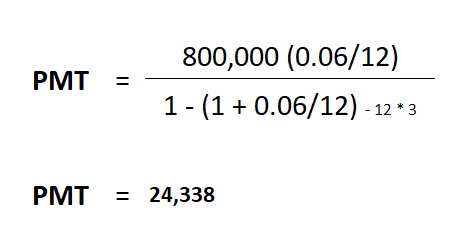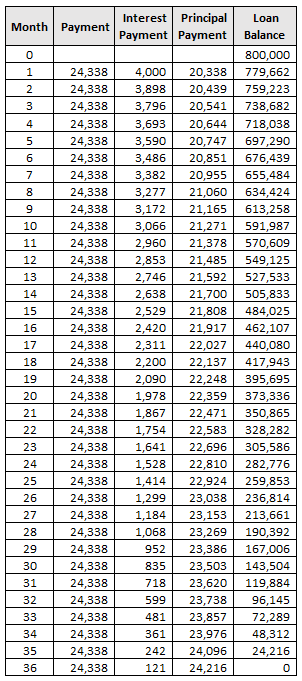# How to compute auto loan and interest?

June 10, 2022 at 3:07PM

Have you calculated the amount of interest you'll have to pay on an auto loan? Maybe you're just relying on the bank's calculations, or maybe you're looking for a website that offers a free auto loan calculator.

When it comes to getting a car loan, you know you want the lowest interest rate and monthly amortization available. However, it is often necessary to understand how the figures are calculated in order to determine whether the loan they are offering is advantageous to you or not.Bank loan office

Before exploring further into how auto loans are calculated, it's important to first understand the factors that go into this calculation:

• Vehicle’s Cost. It is the purchase price of the vehicle when paid in cash. This includes the cost of the vehicle, any relevant taxes, and, in some cases, the registration fee. You must subtract from the overall value the value of any vehicle you intend to trade-in.
• Down Payment. It is an initial payment made when purchasing expensive goods or services. In most cases, a down payment is calculated depending on the rate, for example, a 20% down payment.
• Loan Term. The length of time for a loan to be paid off in full when the borrower is making regular payments. In most cases, the loan term is referred to the number of months to pay.
• Interest Rate. The percentage of the principal amount that the lender charges for the usage of its funds.

## Do the math

To compute the auto loan, here’s the formula:

Auto Loan = Vehicle’s Cost – Down Payment

where;

Down Payment = Vehicle’s Cost x Down Payment Rate

For example, the car’s price is P1,000,000 and the down payment rate is 20%. So, your computation is:

Auto Loan = 1,000,000 – (1,000,000 x 20%)

= 1,000,000 – 200,000

= 800,000

To compute the interest, here’s the formula:

Monthly Interest = Auto Loan Balance x (interest rate / 12)

In the previous example, the initial loan balance is P800,000, and let’s say the bank’s annual interest rate is 6%. The computation would be:

Monthly Interest = 800,000 x (0.06 / 12)

= 4,000

To stay on the same page, take note that an auto loan is an amortizing loan, which means you'll have to pay back the loan amount plus interest on a monthly basis. Your monthly payments are the same and include both the principal and interest. Each payment will be the same, but when you make more, you will pay more toward the principal and less for interest. It's known as the "Declining Balance Method" in accounting since the principal balance decreases as you make more payments.

## How to compute your monthly payment?

As discussed earlier, your monthly payment includes both the principal payment and the interest of the current loan balance. But how to calculate the monthly amortization? We have to use the PMT formula, which is an abbreviation for PAYMENT:where;

P = principal (initial loan amount)

r = interest rate

n = number of payments per year (usually 12 months)

t = time in years

Let us say that the loan is payable for 3 years. To substitute from the previous example,Note: You need to use a scientific calculator in order to arrive at the exact amount of 24,338, regular calculators don’t work.

Alternatively, you can use the PMT formula in MS Excel:

24,338 = PMT( (0.06/12), (3*12), -800000 )

Finally, we arrive at the following repayment schedule using the aforementioned example:As shown in the table, the interest of 4,000 (800,000*(0.06/12)) is applied first to the first month's payment of 24,388, followed by the principal payment of 20,388. The new loan balance is 779,662 after reflecting the principal payment. Every payment repeats the process until the principal balance reaches zero, which exactly occurs on the last payment.

Related Posts

Recent Posts

###### Top 10 Best Websites to Buy and Sell Cars in the Philippines - 2023 Ranking

Since the COVID-19 outbreak began in December 2019, the car-dealing industry has been one of the har...